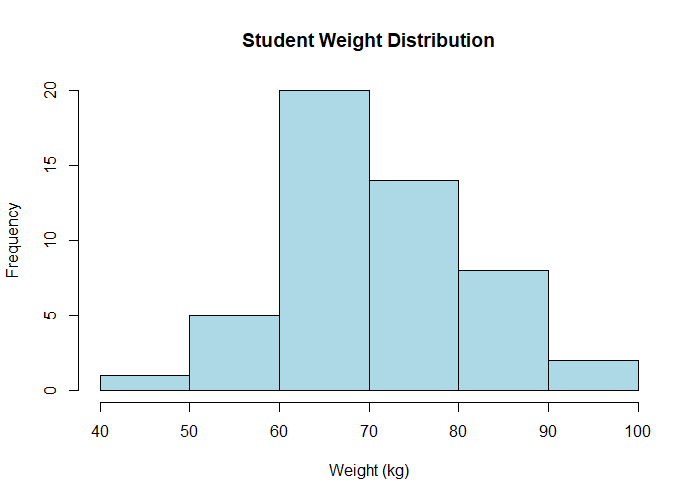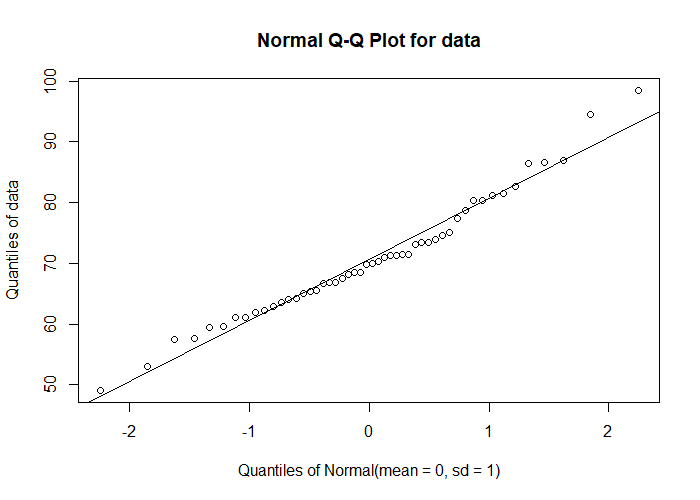# Shapiro–Wilk Test in R

The normal distribution (also known as the Gaussian distribution) is one of the crucial assumption for performing parametric tests such as ANOVA, t-test, regression, and many others.

The Shapiro-Wilk test is used for assessing whether a dataset follows a normal distribution. This test helps to check the assumption of normality.

The Shapiro-Wilk test analyzes the null hypothesis (H0: data comes from a normally distributed population against the alternative Hypothesis (H1: data does not come from a normally distributed population).

To perform the Shapiro-Wilk test of normality in R, you can use the `shapiro.test()` function. The syntax for `shapiro.test()` function looks like this:

``````shapiro.test(data)
``````

Where, `data` is a numeric vector

## Example of Shapiro-Wilk test in R

The following examples demonstrate how to use `shapiro.test()` function for testing the normality assumption in R.

Suppose, we have the following student weight data and would like to check whether this dataset follows normal distribution.

Sample size: Shapiro-Wilk test can be applied on dataset with sample size in between 3 to 5000.

``````# generate random data for student weights
data = rnorm(50, mean = 70, sd = 10)

# perform Shapiro–Wilk Test
shapiro.test(data)

# output
Shapiro-Wilk normality test

data:  data
W = 0.93457, p-value = 0.4943
``````

The p value is greater than significance level of 0.05 (W = 0.9345, p = 0.4943) for student weight data. Hence, we fail to reject the null hypothesis and conclude that student weight data is normally distributed.

In addition to statistical test, you cal also use histogram to visually assess whether the data appears to follow a normal distribution.

Create a histogram,

``````hist(data, main = "Student Weight Distribution", xlab = "Weight (kg)",
ylab = "Frequency", col = "lightblue", border = "black")
``````The shape of the histogram matches the bell shaped curve and suggests that the data is normally distributed.

In addition to histogram, the quantile-quantile (Q-Q) plot could also be used for more rigorous assessment of normality

``````# load package
library(EnvStats)

# Q-Q plot
``````The data closely follows the reference line on Q-Q plot and suggests that data follows approximate normal distribution.

## Subscribe to get new article to your email when published

* indicates required